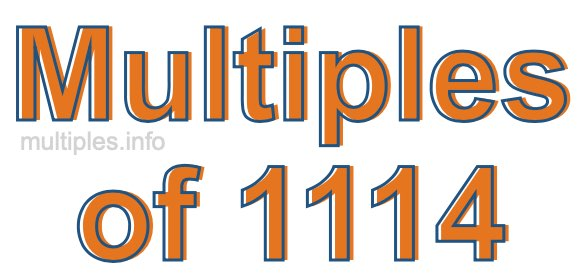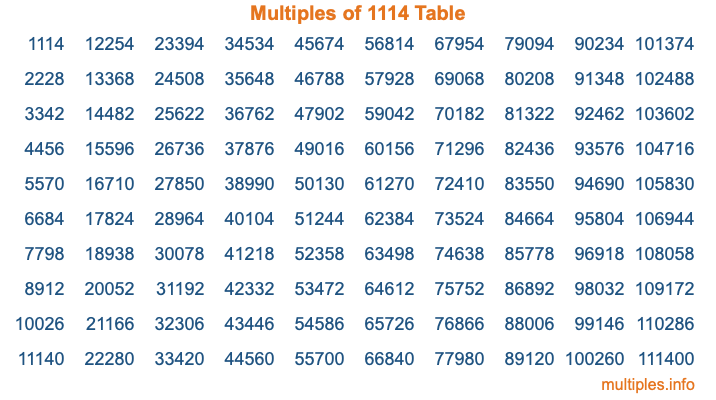Multiples of 1114Welcome to the Multiples of 1114 page. Here we will first teach you everything you will ever need to know about the multiples of 1114, and then give you a study guide summary of everything we taught you to make sure you remember it all. Use this page to look up facts and learn information about the multiples of 1114. This page will make you a multiples of one thousand one hundred fourteen expert!

Definition of Multiples of 1114
Multiples of 1114 are all the numbers that when divided by 1114 equal an integer. Each of the multiples of 1114 are called a multiple. A multiple of 1114 is created by multiplying 1114 by an integer.

Therefore, to create a list of multiples of 1114, you start with 1 multiplied by 1114, then 2 multiplied by 1114, then 3 multiplied by 1114, and so on for as long as you want. Thus, the list of the first five multiples of 1114 is 1114, 2228, 3342, 4456, and 5570. To see a larger list of multiples of 1114, see the printable image of Multiples of 1114 further down on this page. We also have a category where you can choose any nth multiple of 1114.

Multiples of 1114 Checker
The Multiples of 1114 Checker below checks to see if any number of your choice is a multiple of 1114. In other words, it checks to see if there is any number (integer) that when multiplied by 1114 will equal your number. To do that, we divide your number by 1114. If the the quotient is an integer, then your number is a multiple of 1114.

Is  a multiple of 1114?

Least Common Multiple of 1114 and ...
A Least Common Multiple (LCM) is the lowest multiple that two or more numbers have in common. This is also called the smallest common multiple or lowest common multiple and is useful to know when you are adding our subtracting fractions. Enter one or more numbers below (1114 is already entered) to find the LCM.

Check out our LCM Calculator if you need more details about the Least Common Multiple or if you need the LCM for different numbers for adding and subtraction fractions.

nth Multiple of 1114
As we stated above, 1114 is the first multiple of 1114, 2228 is the second multiple of 1114, 3342 is the third multiple of 1114, and so on. Enter a number below to find the nth multiple of 1114.

th multiple of 1114

Multiples of 1114 vs Factors of 1114
1114 is a multiple of 1114 and a factor of 1114, but that is where the similarities end. All postive multiples of 1114 are 1114 or greater than 1114. All positive factors of 1114 are 1114 or less than 1114.

Below is the beginning list of multiples of 1114 and the factors of 1114 so you can compare:

Multiples of 1114: 1114, 2228, 3342, 4456, 5570, etc.

Factors of 1114: 1, 2, 557, 1114

As you can see, the multiples of 1114 are all the numbers that you can divide by 1114 to get a whole number. The factors of 1114, on the other hand, are all the whole numbers that you can multiply by another whole number to get 1114.

It's also interesting to note that if a number (x) is a factor of 1114, then 1114 will also be a multiple of that number (x).

Multiples of 1114 vs Divisors of 1114
The divisors of 1114 are all the integers that 1114 can be divided by evenly. Below is a list of the divisors of 1114.

Divisors of 1114: 1, 2, 557, 1114

The interesting thing to note here is that if you take any multiple of 1114 and divide it by a divisor of 1114, you will see that the quotient is an integer.

Multiples of 1114 Table
Below is an image of the first 100 multiples of 1114 in a table. The table is in chronological order, column by column. The first column has the first ten multiples of 1114, the second column has the next ten multiples of 1114, and so on.The Multiples of 1114 Table is also referred to as the 1114 Times Table or Times Table of 1114. You are welcome to print out our table for your studies.

Negative Multiples of 1114
Although not often discussed or needed in math, it is worth mentioning that you can make a list of negative multiples of 1114 by multiplying 1114 by -1, then by -2, then by -3, and so on, to get the following list of negative multiples of 1114:

-1114, -2228, -3342, -4456, -5570, etc.

Multiples of 1114 Summary
Below is a summary of important Multiples of 1114 facts that we have discussed on this page. To retain the knowledge on this page, we recommend that you read through the summary and explain to yourself or a study partner why they hold true.

There are an infinite number of multiples of 1114.

A multiple of 1114 divided by 1114 will equal a whole number.

1114 divided by a factor of 1114 equals a divisor of 1114.

The nth multiple of 1114 is n times 1114.

The largest factor of 1114 is equal to the first positive multiple of 1114.

1114 is a multiple of every factor of 1114.

1114 is a multiple of 1114.

A multiple of 1114 divided by a divisor of 1114 equals an integer.

1114 divided by a divisor of 1114 equals a factor of 1114.

Any integer times 1114 will equal a multiple of 1114.

Multiples of a Number
Here you can get the multiples of another number, all with the same attention to detail as we did for multiples of 1114 on this page.

Multiples of
Multiples of 1115
Did you find our page about multiples of one thousand one hundred fourteen educational? Do you want more knowledge? Check out the multiples of the next number on our list!